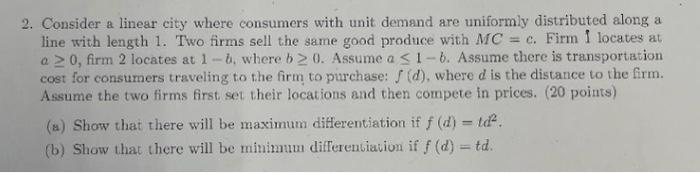Home / Expert Answers / Economics / 2-consider-a-linear-city-where-consumers-with-unit-demand-are-uniformly-distributed-along-a-line-w-pa175

# (Solved): 2. Consider a linear city where consumers with unit demand are uniformly distributed along a line w ...2. Consider a linear city where consumers with unit demand are uniformly distributed along a line with length 1 . Two firms sell the same good produce with . Firm 1 locates at , firm 2 locates at , where . Assume . Assume there is transportation cost for consumers traveling to the firm to purchase: , where is the distance to the frm. Assume the two firms first, set their locations and then compete in prices. (20 points) (a) Show that there will be maximum differentiation if . (b) Show that there will be minimum differentiavion if .

We have an Answer from Expert